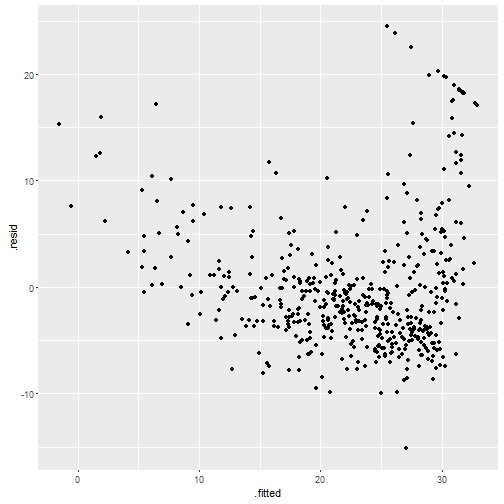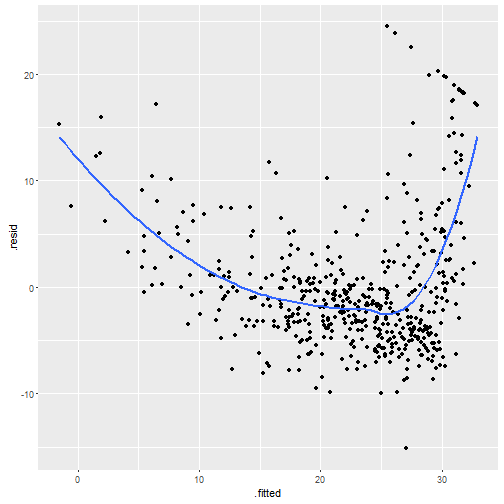# 用broom畫殘差圖(residual plot)

## 例子

``````library(dplyr)
library(ggplot2)
library(broom)
data(Boston, package = "MASS")
bos.fit <- lm(medv ~ lstat, data = Boston)
``````

``````b.res.plot <- bos.fit %>% augment %>%
ggplot(aes(x = .fitted, y = .resid)) + geom_point()
b.res.plot
````````````b.res.plot + geom_smooth(se = FALSE)
``````## 其他函數

`broom`內還有很多方便的函數，像是`tidy()``glance()`，以及可以和`dplyr`一起拿來作拔靴法的`bootstrep`等等。另外David Robinson本身有寫部落格，他的文章也是相當高水準，值得一讀。

Written on November 2, 2016